# Common Core: 6th Grade Math : Solve Real-World and Mathematical Problems by Writing and Solving Equations: CCSS.Math.Content.6.EE.B.7

## Example Questions

← Previous 1 3 4 5 6 7 8 9 10

### Example Question #1 : Solve Real World And Mathematical Problems By Writing And Solving Equations: Ccss.Math.Content.6.Ee.B.7

Solve for: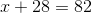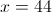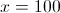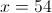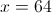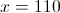Explanation:

Subtract 28 from both sides: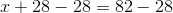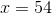### Example Question #2 : Solve Real World And Mathematical Problems By Writing And Solving Equations: Ccss.Math.Content.6.Ee.B.7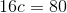What is?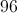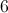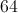Explanation:

Divide both sides by 16: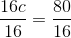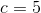### Example Question #3 : Solve Real World And Mathematical Problems By Writing And Solving Equations: Ccss.Math.Content.6.Ee.B.7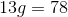What is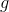?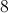Explanation:

Divide both sides by 13: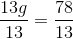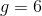### Example Question #1 : Solve Real World And Mathematical Problems By Writing And Solving Equations: Ccss.Math.Content.6.Ee.B.7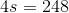What is s?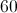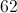Explanation:

Divide both sides by 4: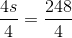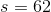### Example Question #5 : Solve Real World And Mathematical Problems By Writing And Solving Equations: Ccss.Math.Content.6.Ee.B.7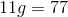What is?Explanation:

Divide both sides by 11: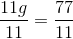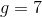### Example Question #6 : Solve Real World And Mathematical Problems By Writing And Solving Equations: Ccss.Math.Content.6.Ee.B.7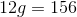What is?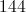Explanation:

Divide each side by: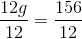### Example Question #7 : Solve Real World And Mathematical Problems By Writing And Solving Equations: Ccss.Math.Content.6.Ee.B.7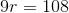What is?Explanation:

Divide each side by: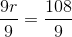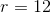### Example Question #8 : Solve Real World And Mathematical Problems By Writing And Solving Equations: Ccss.Math.Content.6.Ee.B.7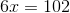What is?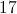Explanation:

Divide each side by: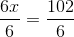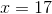### Example Question #9 : Solve Real World And Mathematical Problems By Writing And Solving Equations: Ccss.Math.Content.6.Ee.B.7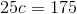Explanation:

Divide each side by 25: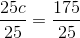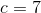### Example Question #10 : Solve Real World And Mathematical Problems By Writing And Solving Equations: Ccss.Math.Content.6.Ee.B.7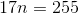Explanation:

Divide each side by: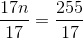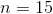← Previous 1 3 4 5 6 7 8 9 10

### All Common Core: 6th Grade Math Resources